# intro to c Caras → XCO D Question 6 10 pts The program below is intended...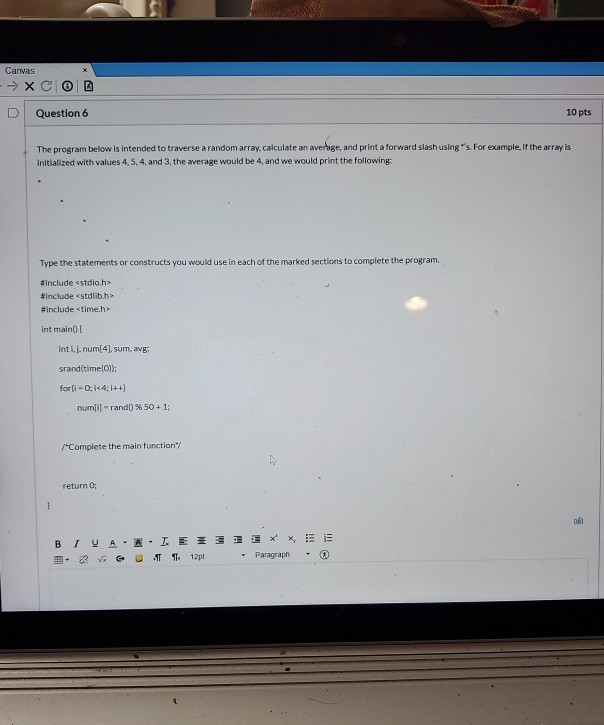intro to c

Caras → XCO D Question 6 10 pts The program below is intended to traverse a random array, calculate an average and print a forward slash usings. For example, if the array is Initialized with values 4, 5, 4, and 3, the average would be 4, and we would print the following Type the statements or constructs you would use in each of the marked sections to complete the program #include <stdio.h> #include <stdlib.h> #include <time.h> int maino inti.j. num, sum, avgi srand(time(0); forli-0;i<4;i++) num] -rand%50+1; "Complete the main function/ return BIVAA. I. E31. XX.EE .. ? V U 1 2p Paragraph .

Note: if you want minimum number need to change num[i] = rand() % 50 + 1; to num[i] = rand() % 5 + 1;

File: intro.c

#include<stdio.h>
#include<stdlib.h>
#include<time.h>
int main()
{
int i, j, num, sum, avg;
srand(time(0));
for(i=0; i<4; i++)
num[i] = rand() % 50 + 1;

sum = num + num+num+num;//calculate sum
avg = sum / 4; //calculate average
printf("\nSum: %d", sum);//display sum
printf("\nAverage: %d\n", avg);//display average
for(i=0;i<avg;i++)//display *
{
for(j=0;j<i;j++)
{
printf("\t");
}
printf("*\n");
}

return 0;
}

Screen: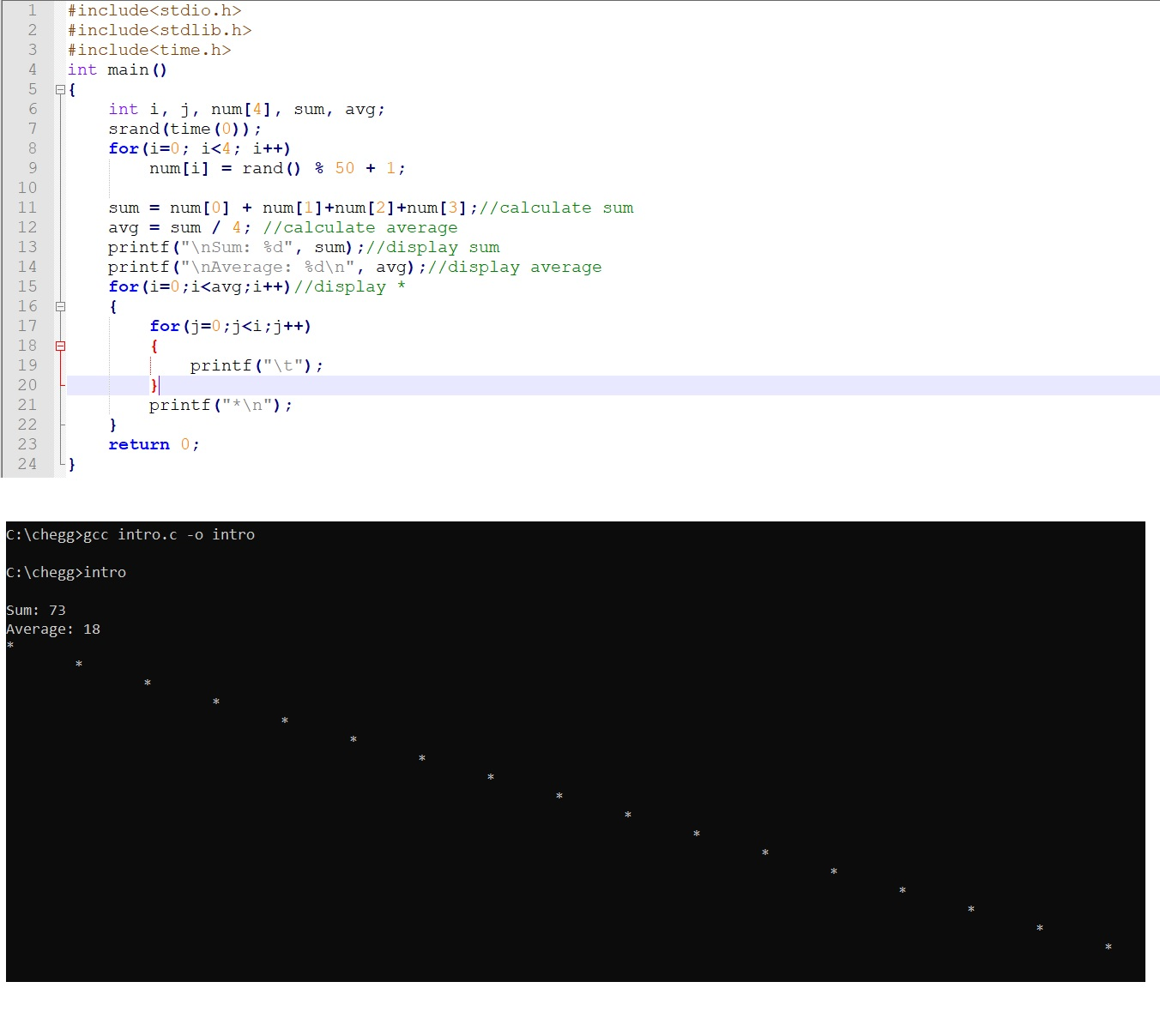##### Add Answer to: intro to c Caras → XCO D Question 6 10 pts The program below is intended...
Similar Homework Help Questions
• ### C++ Program - Arrays- Include the following header files in your program:     string, iomanip, iostream Suggestion:...

C++ Program - Arrays- Include the following header files in your program:     string, iomanip, iostream Suggestion: code steps 1 thru 4 then test then add requirement 5, then test, then add 6, then test etc. Add comments to display assignment //step 1., //step 2. etc. This program is to have no programer created functions. Just do everything in main and make sure you comment each step. Create a program which has: 1. The following arrays created:                 a. an array...

• ### Coin Toss Program

I have this problem to write in C++ class"Write a program that has a function prototype before main and an implementation of the function after main. The function to be implemented is a coin tosssimulation using the random number generator. The function should return 1 or true 50% of the time and 0 or false 50% of the time. Use srand and the system time tomake the program run differently each time. (srand(time(NULL));). Keep track of the number of head...

• ### I need Help PLZ. I can't solving this in C program Given is a C program count_primes.c, which is an array Generates 10000 six-digit random numbers and then determines the number of primes in that...

I need Help PLZ. I can't solving this in C program Given is a C program count_primes.c, which is an array Generates 10000 six-digit random numbers and then determines the number of primes in that array. It also measures the time that elapses during the calculation and returns it to the console along with the number of primes it finds. (a) Rewrite the program so that N threads are used to count the primes in the array. Each thread is...

• ### Learn the example C program in Folio consisting of three files (a.c, a.h, main.c) and complete this exercise. You need t...

Learn the example C program in Folio consisting of three files (a.c, a.h, main.c) and complete this exercise. You need to understand function, pointer and the selection sort algorithm. Write a C program that consists of three files mysort.h, mysort.c and myMain.c. Below is the mysort.h prototype #include <stdlib.h> #include <stdio.h> void generateNums(int *myarr, int len); void sortNums(int *myarr, int len); mysort.h must contain only the function declarations (prototypes) listed above generateNums function should generate len random integers in the...

• ### c/c++ This is a simple debugging question. The program (as written) tries to add up the...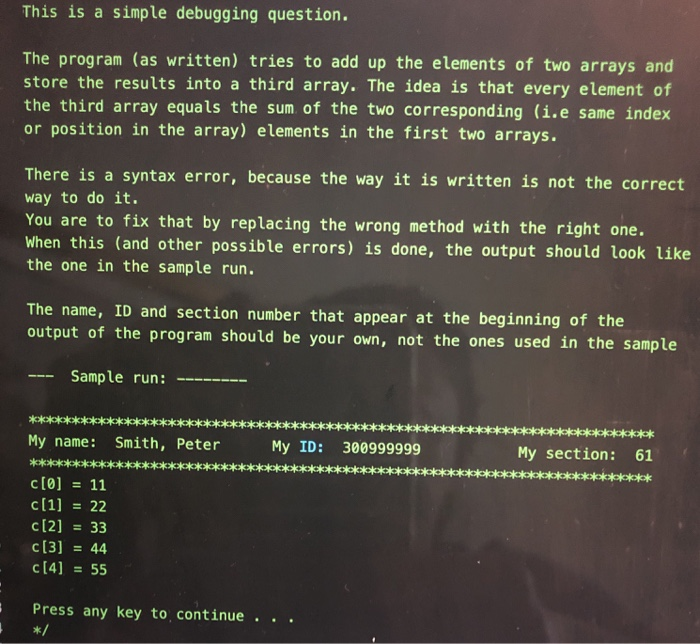c/c++ This is a simple debugging question. The program (as written) tries to add up the elements of two arrays and store the results into a third array. The idea is that every element of the third array equals the sum of the two corresponding (i.e same index or position in the array) elements in the first two arrays. There is a syntax error, because the way it is written is not the correct way to do it. You are...

• ### Given below is a partially completed C program, which you will use as a start to...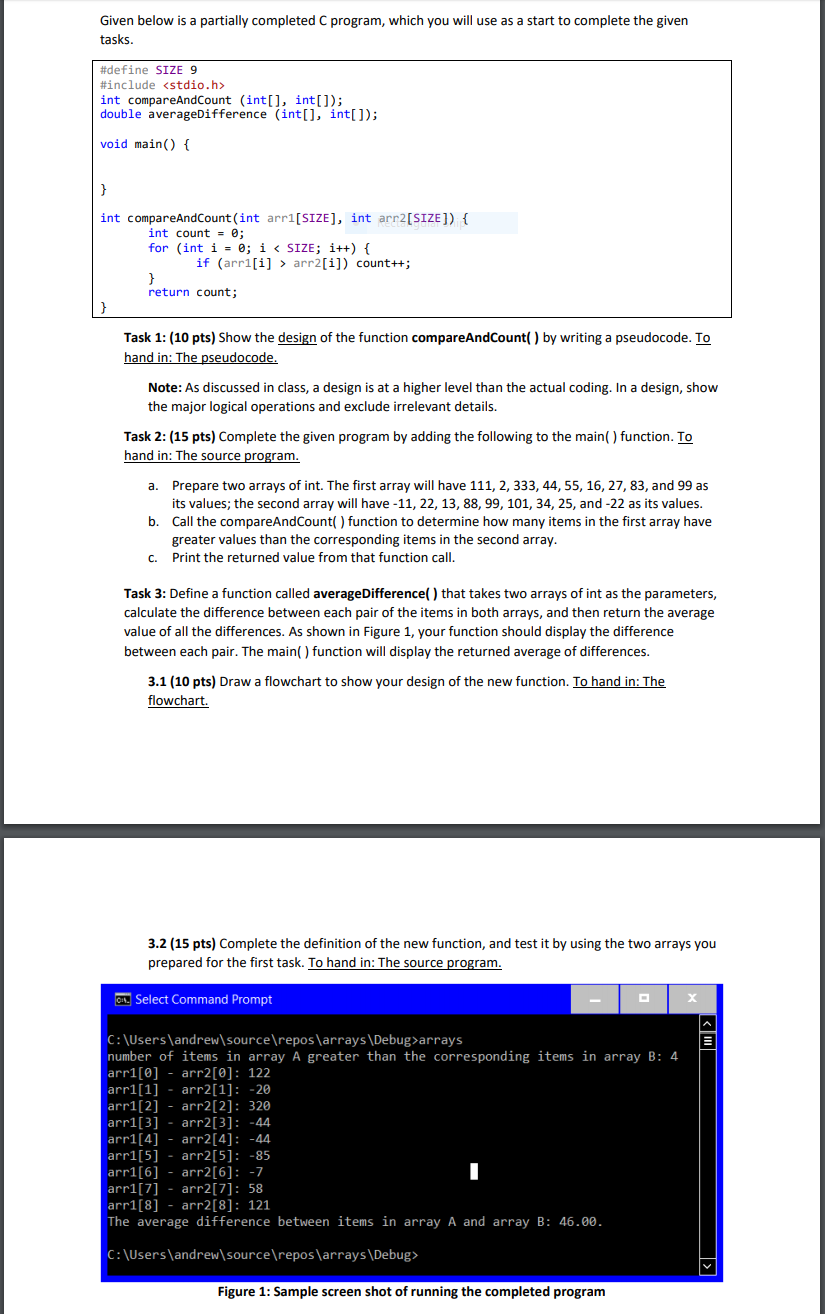Given below is a partially completed C program, which you will use as a start to complete the given tasks. #define SIZE 9 #include <stdio.h> int compareAndCount (int[], int[]); double averageDifference (int[], int[]); void main() { } int compareAndCount(int arr1[SIZE], int arr2[SIZE]) { int count - @; for (int i = 0; i < SIZE; i++) { if (arri[i] > arr2[i]) count++; return count; Task 1: (10 pts) Show the design of the function compareAndCount() by writing a pseudocode. To...

• ### ***Please complete the code in C*** Write a program testArray.c to initialize an array by getting...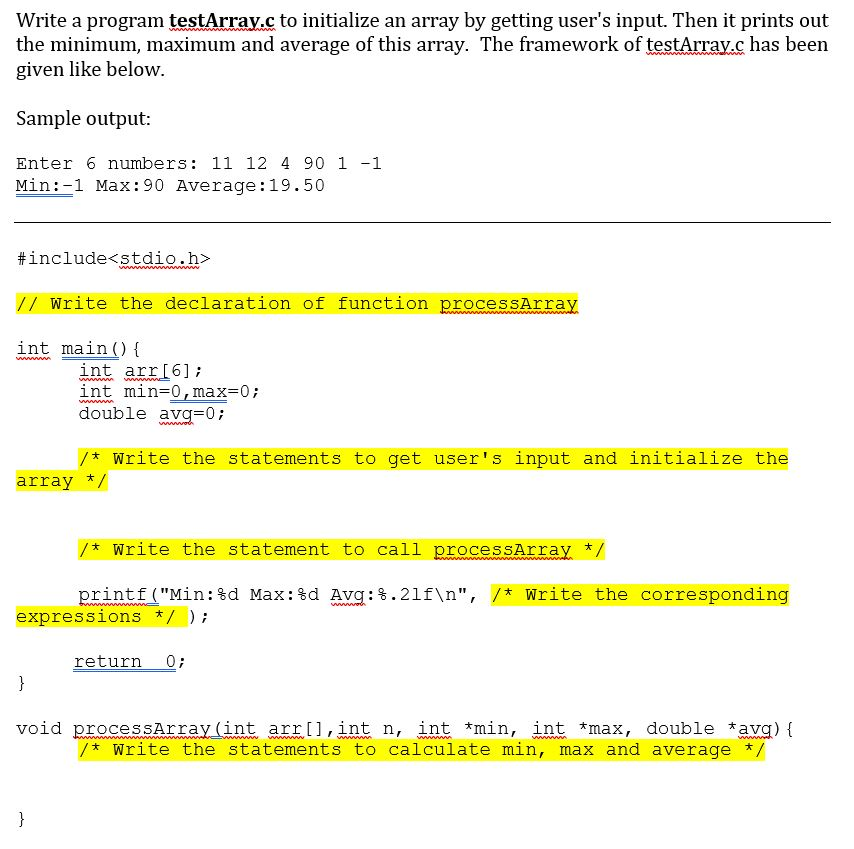***Please complete the code in C*** Write a program testArray.c to initialize an array by getting user's input. Then it prints out the minimum, maximum and average of this array. The framework of testArrav.c has been given like below Sample output: Enter 6 numbers: 11 12 4 90 1-1 Min:-1 Max:90 Average:19.50 #include<stdio.h> // Write the declaration of function processArray int main) I int arrI6]i int min-0,max-0 double avg=0; * Write the statements to get user's input and initialize the...

• ### Use the C programming language to complete #include <stdio.h> #include <stdlib.h> #include <float.h> // Declare Global...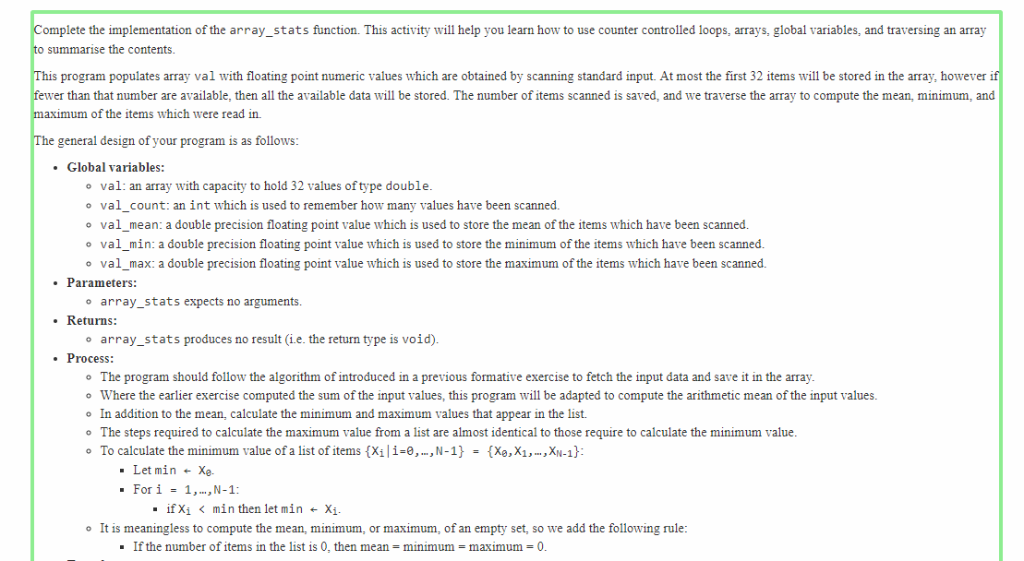Use the C programming language to complete #include <stdio.h> #include <stdlib.h> #include <float.h> // Declare Global variables here. void array_stats() { // Insert your solution here. } #include <stdlib.h> #include <time.h> int main() { // Simulate the test setup process. srand( time( NULL ) ); for ( int i = 0; i < 32; i++ ) { val[i] = rand(); } val_count = rand(); val_mean = rand(); val_min = rand(); val_max = rand(); // Call submitted code. array_stats(); // Display...

• ### C++ PROGRAM You MUST complete the hilo game, funny face and magic8ball game to earn a...

C++ PROGRAM You MUST complete the hilo game, funny face and magic8ball game to earn a 100. Instructions are in the program in the respective functions. #include <iostream> #include <string> #include <iomanip> #include <cmath> #include <cstdlib> #include <ctime> using namespace std; void printmenu(); void hilo(); void magic8ball(); void funnyface(); string secretword = "hello"; //you can delete secretword from here and then //have a user enter the word in the function int main() { int choice, notquit = 1; while (notquit)...

• ### Question 1 In the following incomplete C program: #include stdlib.h> #include <stdio.h> int main () { int i, n, max; int array; ... } return 0; } an array of random int values is populat...

Question 1 In the following incomplete C program: #include stdlib.h> #include <stdio.h> int main () { int i, n, max; int array; ... } return 0; } an array of random int values is populated with n random values. Write only the code to find the location of the maximum value in the array. Question 2 Follow these instructions in your Linux account: 1. Create a file called data.txt in the root folder in your account. 2. Create a new...

Free Homework App